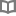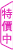本草綱目中的家庭保健智慧 - 焦亮 - BOOKWALKER中文電子書

本草綱目中的家庭保健智慧

焦亮

焦亮 筆名焦木，河北保定人，出生於中醫世家，自幼耳濡目染，對中醫養生具有濃厚興趣，在求學期間便廣獵中醫學知識，加強自身的醫學專業素養，畢業後一直從事中醫養生文化的宣傳工作，工作期間曾利用空餘時間拜訪專家名醫，在各個國醫大師的指導下，醫學理論水平得到了顯著地提高，並積累了大量獨具特色的養生方法，這些方法簡單、有效，備受廣大使用者追捧。目前已編輯出版了《大國醫I、II》、《不生病的智慧大全集》、《黃帝內經養生智慧大全集》等多部養生作品。

• 放入購物車
•試閱

• 放入購物車
•試閱

• 放入購物車
•試閱

• 放入購物車
•試閱

• 放入購物車
•試閱

• 放入購物車
•試閱

• 放入購物車
•試閱

• 放入購物車
•試閱

• 放入購物車
•試閱

• 放入購物車
•試閱

• 放入購物車
•試閱

• 放入購物車
•試閱

• 放入購物車
•試閱

• 放入購物車
•試閱

• 放入購物車
•試閱

• 放入購物車
•試閱

• 放入購物車
•試閱

• 放入購物車
•試閱

• 放入購物車
•試閱

• 放入購物車
•試閱

• 放入購物車
•試閱

• 放入購物車
•試閱

• 放入購物車
•試閱

• 放入購物車
•試閱

• 放入購物車
•試閱• 放入購物車
•試閱

• 放入購物車
•試閱

• 放入購物車
•試閱

• 放入購物車
•試閱

• 放入購物車
•試閱

• 放入購物車
•試閱

• 放入購物車
•試閱

• 放入購物車
•試閱

• 放入購物車
•試閱

• 放入購物車
•試閱

• 放入購物車
•試閱

• 放入購物車
•試閱

• 放入購物車
•試閱

• 放入購物車
•試閱

• 放入購物車
•試閱

• 放入購物車
•試閱

• 放入購物車
•試閱

• 放入購物車
•試閱

• 放入購物車
•試閱